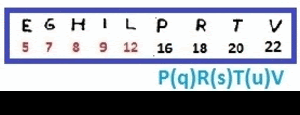## HERVPILTG : LEIGH PRTV## quinta-feira, 22 de setembro de 2016

### THE 17X17 Z340 CODE = Z289 CIPHER .

I noticed that, by eliminating the non-alphabetic symbols of the first line leaving only the alphabetic symbols left (see image below) the sequence

H E R V P I L T G

After that eliminating such letters (51) ( H E R V P I L T G) of Z340 code as a whole remaining 289 symbols, which form perfectly 17 rows and 17 columns.
What I found interesting was to have appeared the number 17, which is known to all who follow the case of the infamous zodiac, is closely linked to both the Z340, as the z 408 and partially to the z32.
And besides, after elimination of such symbols alphabetic (H E R V P I L T G), remains in this new cipher 54 symbols, ie the same number of symbols used in Z408 (decrypted)

below the "Z 289" in image and text (which allows to work in)

340 HERVPILTG

"new cipher"

4 ZKDecrypto

> Ð Ì ^ Ë ² ± Ä N Ð + B ¢ · O º D
W y • < » K Æ £ B Ÿ „ Ã M + u Z W
¢ £ · ¤ J S Ð Ð ½ ^ Ì ¾ » ´ Ð O +
+ K ± ¸ ¼ M + ¤ Ô Ê Ä µ F + ˆ ³ Ë
/ Ð ¾ ^ F Ì O - » Ä C Ë F > ± D ¢
· µ + K Ñ º ƒ ± u Ã X • ¤ ¢ ± J Æ
Ê · O + ¸ N y ¤ + ¹ ¼ Ä < M + Â +
Z ± F B Ã Ÿ A ° ³ K - ¤ Ì u + ^ J
+ O Ð ½ < F B Ÿ - u + / µ Ô D y B
Ð Â M K O ± < Ã Ì J » µ ³ M • + ˆ
B F ¤ ° ¼ S Ÿ · + N µ F B Ã ¢ ƒ ¾
Ì F N ^ Æ µ ± ³ Â • Ã ³ Ô + + Ÿ B
X ² » „ ³ ¼ C > u Z µ - + Ã • ´ ¤
B K ¢ O Ð ^ • Æ M Ñ ± Ã + ² ° C <
+ F Ì W B £ + + £ W C ¤ W Ã O S /
¢ £ Ð F Ë Ä W < ½ Ô B ¸ y O B » -
C Ã > M D N Ð Ë S ¤ Z O ¾ A K ƒ +

Hello good people and some friends. :D

I'm working on "Z 289" And I'm using various ways on the cipher.
In this case I set spiral center (symbol -) to the last end to the vertical. I have built several models around.
Below the title of mere example.

I LOST SSTOON AND THE
L I DO FALL ESSLEDRDE
RINPNVO YOU DEDOBUT
UYFELDLITYOFTYALD
THIELALITYTDTFRAS
IYMPIDENOTNLHISHE
TONALATARTLSISTIT
HELL ARE SEES OR THAN
BEEN TO SANDLORTDR
OPELEDTASSEDASISU
NINORDEDSTOOSIMIL
ATELLLYAINRLRATER
ESEATPOLIAILAYSLI
INYA ILLSLAVE THE SL
ININTERSEEALASEM
ETASHINTLELYRESOI
ILYFOLDSA IT I LEAST

spiral code

ŸFC>ÊÐ¢+Cuµ½J^+ƒD
<ŸËCÄOM¾WÐÐ<ÂËBKW
BN•¼µ„u±£ˆKW^²XA+
AºÄ³F^Ô·+±£Äy±Ì¾Ë
yƒ¤ÆF°M¤+±yË+ÄBO¢
¤±/¼¤KÃJ£+•FƒNÐZÆ
+uµO<½+SB+<ÐŸÐ+¤+
¹ÃF<ÌBÃÐWD»£´+ZSJ
KX³Ãµ+²ÐÌ•KFCBÊËB
u¼Ã¸³K+½¢¢ÆKS¢·Ðˆ
J-•ÑB^Ã^»+£²»·/N¾
Ì+ÂFÔ¸±ÌN•BFBO+DB
Ã»³Ì+¼Ñ¾¤°ŸFOº¢M¤
Ÿµ±O-MM»MO„ÂyZW><
°Nµ-µ+Æ´ÐÃÃO¸O>Ã/
³+S»Z¤•+FWM±B³ÐC-
-¸±ÄuÔ^>O¤+·ÔDÌ¢+

PRTV

The "final sequence" PRTV, are alphabetic equidistant symbols in order sequence, there is therefore, in theory, a symmetry both in sequence "EGHIL" which demonstrated already through the Archimedes spiral (which forms a name), as in this sequence (PRTV). Look at the image below.

I made a picture of a mounting scheme of my theoretical Z 289, in which, as I said in the beginning, is only the elimination of the sequence of alphabetical symbols of the first line of the 340 original cipher, which results in 289 symbols, or 17x17 rows and columns formed by 54 different symbols, as well as z408.
I found this interesting image as the symbol + was the centro.Mas there may be other ways, if it has or will have some sense someday, but finally, the Z340 was already turned over all the ways without success until now.

sharing

HelloThe assembly of the cipher z289 to illustrate see image below in which fits into an official target shooting 4 air rifle), I'm turning the symbols for the circle track (which are 8 in total, and the ninth is the symbol + center) which as I said, by different paths.
I noticed that each band occupies in arithmetic progression - 8 (which would occur in any symmetric cipher) the following progression
symbols per track
1 = 64
2 = 56
3 = 48
4 = 40
5 = 32
6 = 24
7 = 16
8 = 08
9 = 1 (+)

So I do rearrangements with birth dates, posting dates of the letters, dates of crimes attributed to the infamous z, etc.(Moving independent tracks as if they were combinations to open a safe)
Finally, it may be a waste of time, which is very likely, but I enjoy it (for now until I get sick of it), I share because I believe that this is the goal of this community. Exchange of ideas, learning and other theories.

MarceloYou can follow the "evolution" of ideas around the cipher "Z289", the topic I did in zodiackillersite.com